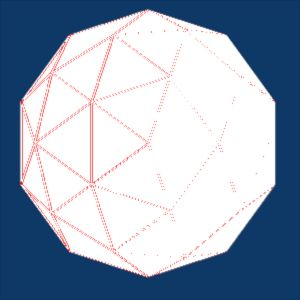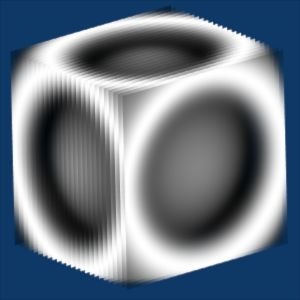# The official WIRE.py texture thread

http://wiki.blender.org/bin/view.pl/Blenderdev/PyTexture

pytexwire08.jpg ==> e=0.01pytexwire08.jpg ==> e=60.0``````#-----------
# wire.py pytex
#    jms 09/2005
#
#  pytexwire08.jpg ==&gt; e=0.01
#  pytexwire10.jpg ==&gt; e=0.01    transparent
#  pytexwire11.jpg ==&gt; e=150.0   transparent
#  pytexwire09.jpg ==&gt; e=60.0
#----------
import Blender
from Blender import Mathutils

tex = Blender.Texture.Get("Tex")
texvec = Mathutils.Vector(tex.getPytexCoords())
e=0.01
try :
if Blender.texvec2 :
vecnor=Mathutils.CrossVecs(Blender.texvec2,texvec)
Blender.texvec2=texvec
A=Mathutils.AngleBetweenVecs(Blender.vecnor,vecnor)
if abs(Blender.A-A)&gt;=e:
r = 1.0#/abs(Blender.A-A)
g = 0.0
b = 0.0
tex.setPytexColour(r, g, b)
print Blender.A-A
else:
r = 1.0
g = 1.0
b = 1.0
tex.setPytexColour(r, g, b)
Blender.vecnor=vecnor
Blender.A=A
else :
Blender.texvec2=texvec
except :
Blender.A=0.0
Blender.texvec2=texvec
Blender.vecnor=Mathutils.CrossVecs(Blender.texvec2,texvec)
``````

Nice to see a PyTex topic pop up already. I haven’t had a chance to change to wiki doc yet, but I highly recommend you no longer use Blender.Texture.Get for pytex instead:

``````tex = Blender.Texture.GetCurrentPytex()
``````

This allows a couple of things :
a) inherent error checking
b) a pytex script can be linked to any number of textures, instead of being limited by the value given the Blender.Texture.Get.
c) its several times faster than Blender.Texture.Get, and this can climb to 10-15 times faster if there are a lot of textures in the scene.

I hope to see more pytex scritps begin shared around.

timmeh

: btw nice work jms, looks goodSorry, but now, with this, blender fails (yesterday blenderpytex version, and
it fails witout too…)

Nice.

I was just thinking, if you want to play with PyTex, how’s this for an idea for a cool experiment…

Create a load of falling empties (keyframe it, bake a physics simulation, particle system or whatever). Then define your texture plane (that has been subdivided multiple times) at a certain height. Using python and Pytex, create an animated texture (or more specifically a displacement map) that creates ripples everytime an empty passes through the texture plane!This sort of thing would be so very cool for simulating rain falling into a lake or something. Essentially it is like the ripple texture plugin except that it is triggered correctly.

Problem is getting some fast ripple code.

What do you think?

Koba

Use of a bunch of empties is a good idea but Pytex seems to work in a 3d space and not only on a plane .

Well…

The idea is that you use normal blender python to find the location of the empties in three dimensional space. When the z-value of one of the coordinates drops below a certain value (the height of the plane), python reads off the other two coordinates in world space and somehow (I don’t know how) ensures that on the next frame a ripple is generated at the point the empty crossed the plane.

I know that Python works in 3D but this is the simplest implementation I can think of.

Koba

for the moment , i just can do this :

``````import Blender
from  math  import *

tex = Blender.Texture.Get("Tex")
texvec = tex.getPytexCoords()

b,r,g=1.0,1.0,1.0
def col_func2(tex, c):
global b,r,g
r=g=b=0.5+0.5*sin(abs(((c)**2)+((c))**2+((c))**2)**.5*10.0)
tex.setPytexColour(r, g, b)
tex.setPytexAlpha(r)

col_func2(tex, texvec)

``````